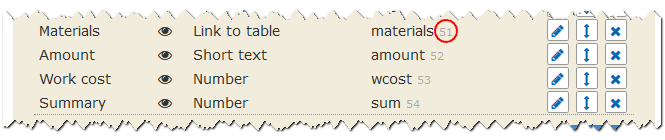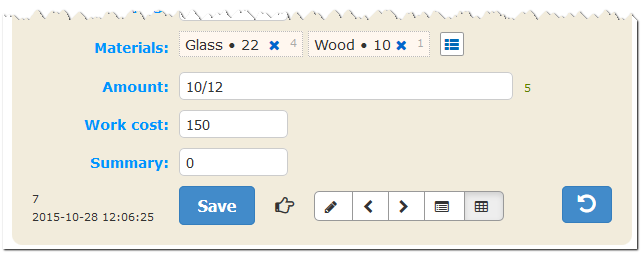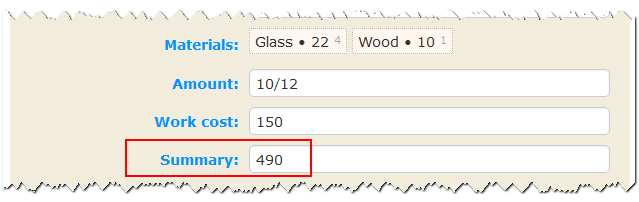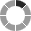# Tracking changes in records and additional PHP processing

```function update_action( \$id ) {
\$db = DB::GetInstance();
}```

The function from the shown example effectively does nothing, but it is called upon every successful adding or changing of any record in the table that has the action alias. In the code of this function you can write any PHP code. To access the database you can use methods from the lib/safemysql.class.php and lib/extmysql.class.php modules. You can describe functions directly in conf.inc.php or include separate files there.

Let’s take a look at this specific example. Suppose, the handmade table accounts some works done and is linked to the materials table that stores production costs per unit. In the handmade table you can select several materials for every work and set the given amount of materials actually spend separating them with '/'.Let’s implement a special function to automatically fill the Summary field to save some time on manual calculation of funds spent on materials and costs of the work in total.

```function update_handmade( \$id )
{
\$db = DB::GetInstance();
\$item = \$db->getrow("select amount, wcost, m.cost as cost from `handmade` as t
left join materials as m on m.id = t.materials
where t.id=?s", \$id );
\$actions = explode( '/', \$item['amount'] );
\$list = \$db->getall("select m.cost from ?n as t
left join materials as m on m.id = t.idmulti
where t.idcolumn=?s && t.iditem=?s order by t.id", ENZ_ONEMANY, 51, \$id );
\$sum = (int)\$item['wcost'] + ( isset(\$actions ) ? \$item['cost']*\$actions : 0 );
\$i = 1;
foreach ( \$list as \$il )
{
if ( isset( \$actions[\$i])) {
\$sum += \$il['cost'] * \$actions[\$i];
}
\$i++;
}
\$db->update( 'handmade', array( 'sum' => \$sum ), '', \$id );
}```

The result of this function is automatically calculated value of the Summary field.Note that this possibility if quite useless for an average user. At the bare minimum he or she must possess knowledge of the PHP programming language and the MySQL query language. The user must also understand the structure of the database and figure out how the information is stored.